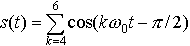EECS20N: Signals and Systems

# Fourier series coefficients

Consider an audio signal given by

s(t) = sin(440× 2π t) + sin(550 × 2π t) + sin(660× 2π t).

This is a major triad in a non-well-tempered scale. The first tone is A-440. The third is approximately E, with a frequency 3/2 that of A-440. The middle term is approximately C sharp, with a frequency 5/4 that of A-440. It is these simple frequency relationships that result in a pleasant sound. We choose the non-well-tempered scale because it makes it much easier to construct a Fourier series expansion for this waveform.

To construct the Fourier series expansion, we can follow these steps:

• Find p, the period. The period is the smallest number p such that s(t) = s(t − p). To do this, note that

sin(2πft) = sin(2πf (t − p))
if f p is an integer. Thus, we want to find the smallest p such that 440p, 550p, and 660p are all integers. Equivalently, we want to find the largest fundamental frequency f0 such that 440/ f0, 550/ f0, and 660/ f0 are all integers. Such an f0 is called the greatest common divisor of 440, 550, and 660. This can be computed using the gcd function in Matlab. In this case, however, we can do it in our heads, observing that f0 = 110.
• Find A0, the constant term. By inspection, there is no constant component in s(t), only sinusoidal components, so A0 = 0.
• Find A1, the fundamental term. By inspection, there is no component at 110 Hz, so A1 = 0.
• Find A2, the first harmonic. By inspection, there is no component at 220 Hz, so A2 = 0.
• Find A3. By inspection, there is no component at 330 Hz, so A3 = 0.
• Find A4. There is a component at 440 Hz, sin(440× 2π t). We need to find A4 and φ 4 such that A4cos(440× 2π t +φ 4) = sin(440× 2π t). By inspection, φ 4 = - π /2 and A4 = 1.
• Similarly determine that A5 = A6 = 1, φ 5 = φ 6 = - π /2, and all other terms are zero.
Putting this all together, the Fourier series expansion can be writtenwhere ω 0 = 220π.

Clearly this method for determining the Fourier series coefficients is tedious and error prone, and will only work for simple signals. We will see much better techniques.

## Exercise

Determine the fundamental frequency and the Fourier series coefficients for the well-tempered major triad,

s(t) = sin(440 × 2π t) + sin(554 × 2π t) + sin(659 × 2π t).